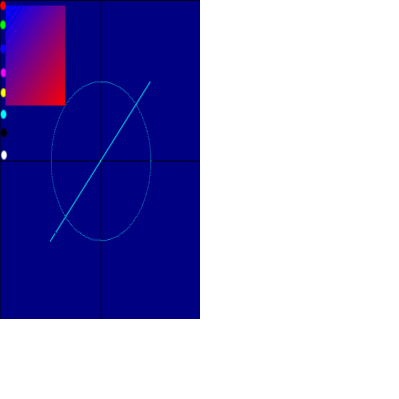# Affine Transformation

## Scale, shear, translate- by matrix

This was written to make sure I understood how to transform images by geometric matrix multiplications. It is ridiculously slow, and with no interpolation/aliassing can produce very patchy quality results.### Asymmetric scaling and shearing results-```
'   **********      Affine transforms of 2D figures. GUI5b   Jan  2012    **************
'                                       uses ( corrected)
'   **********      MaTricks11   v15     tenochtitlanuk      June 2011    **************

'mainwin 80 30
nomainwin

WindowWidth  =840
WindowHeight =660

graphicbox #w.gb1,  10,  50, 400, 400
graphicbox #w.gb2, 420,  50, 400, 400

texteditor  #w.te,  10, 480, 550,  80

global x, y

Open "gdi32" for DLL as #gdi
gopen =1

open "Affine transforms" for window as #w

#w.gb1 "down"
#w.gb2 "down ; fill black ; size 2 ; flush"

#w "trapclose [quit]"

#w.te "!font courier_new 10"

sze =1

hW = hwnd( #w.gb1)
open "user32" for DLL as #user
callDLL #user, "GetDC", hW as long, hDC as long
close #user

#w.gb1 "down ; drawbmp tempscr 0 0"
#w.gb1 "flush ; size sze"

MatM\$ ="3,3,      0.9,0,0,    0,0.9,0,      0,0,1"
'MatM\$ ="3,3,      0.7071,-0.7071,0,     0.7071,0.7071,0,     0,0,1"
call DisplayMatrix MatM\$

for x =1 to 400 step sze
for y =1 to 400 step sze
xx =( x -200) /100
yy =( y -200) /100
MatA\$ ="3,1,       " +str\$( xx) +"," +str\$( yy) +",1"
'MatM\$ ="3,3,       " +str\$( 1 +( y -200) /400)+ ",0,0,      0,1,0,      0,0,1"
MatP\$ =MatrixMultiply\$( MatA\$, MatM\$)
xtr   =GetTerm( MatP\$, 1, 1)
ytr   =GetTerm( MatP\$, 2, 1)
call plot xtr,  ytr, 2, getCol\$( PixelLong( hDC, x, y))
'print x, y, xx, yy, longPixel, xtr, ytr,
'call DisplayMatrix MatM\$
scan
next y
next x

#w.gb2 "flush"

wait

[quit]' h\$
if gopen =1 then close #gdi
close #w
end
'end sub

function getCol\$( color)
blue    =int( color / 2^16)
green   =int( ( color- blue *2^16) /2^8)
red     =color -blue *2^16 -green *2^8
getCol\$ =str\$( red) +" " +str\$( green) +" " +str\$( blue)
'print getCol\$
end function

Function PixelLong( hDC, xVar, yVar)
CallDLL #gdi, "GetPixel", hDC as Ulong, xVar as uLong, yVar as uLong, PixelLong as Long
PixelLong  =( PixelLong + 16777216) mod 16777216
End Function

Function hDC(handle)
CallDLL #user32, "GetDC", handle as Ulong, hDC as Ulong
End Function

Sub ReleaseDC hW, hDC
CallDLL#user32,"ReleaseDC", _
hW as Ulong, _
hDC as Ulong, _
result as Long
End Sub

sub plot x, y, screen, cl\$
sx =200 +int( 100 *x)
sy =200 +int( 100 *y)
if screen =1 then
#w.gb1 "color "; cl\$; " ; set "; sx; " "; sy
else
#w.gb2 "color "; cl\$; " ; set "; sx; " "; sy
end if
end sub

function GetTerm( in\$, i, j)    '   Return element( i, j) first =w column#, second =h row#
w =eval( word\$( in\$, 1, ","))
h =eval( word\$( in\$, 2, ","))
if (w BRow) then notice "Matrix dimensions unsuitable. Not conformable": end
n\$ =str\$( AColumn); ","; str\$( ARow); ","
for AnsColumn =1 to AColumn
for AnsRow =1 to ARow
trm =0
for i =1 to BRow '3
j1   =GetTerm( inA\$, i,      AnsRow)
j2   =GetTerm( inB\$, AnsColumn, i     )
trm  =trm + j1 *j2
next i
n\$ =n\$ +str\$( trm) +","
next AnsRow
next AnsColumn
MatrixMultiply\$ =left\$( n\$, len( n\$) -1) '   ############## trailing ',' to remove
end function

'"Matrix G is reflect x coords across y =0"
'MatG\$ ="3,3,       -1,0,0,     0,1,0,     0,0,1"

'"Matrix H is rotate 45 degrees round origin"
'MatH\$ ="3,3,      cos gama,-sin gamma,0,sin gamma,cos gamma,0,0,0,1"
'MatH\$ ="3,3,      0.7071,-0.7071,0,     0.7071,0.7071,0,     0,0,1"

'"Matrix I is translate +1 +1"
'MatI\$ ="3,3,       1,0,0,     0,1,0,      xt,yt,,1"
'MatI\$ ="3,3,       1,0,0,     0,1,0,      1,1,1"

'Matrix J is scale up 50% along x-axis
'MatJ\$ ="3,3,      1.5,0,0,      0,1,0,      0,0,1"

'Matrix K is scale up 50% along y-axis
'MatK\$ ="3,3,      1,0,0,      0,1.5,0,      0,0,1"

'Matrix M is scale up ( zoom)
'MatM\$ ="3,3,      1.5,0,0,    0,1.5,0,      0,0,1"

'Matrix L is shear parallel to x-axis. NB needs evaluating inside the loop.
'MatL\$ ="3,3,      "+ str\$( 1 +abs( y))+ ",0,0,      0,1,0,      0,0,1"

tenochtitlanuk ------- JohnF ------ April 2020 ------ mr dot john dot f at gmail.com
```